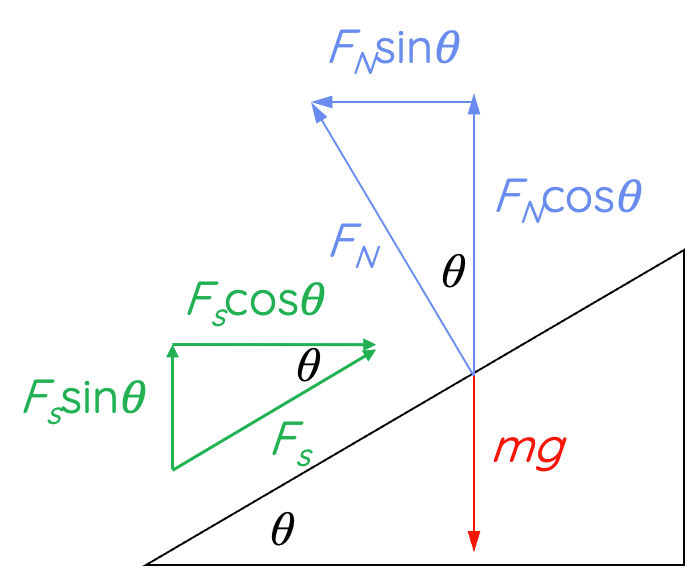# Circular Motion on Banked Surfaces With Friction

This topic is part of the HSC Physics course under the section Circular Motion.

### HSC Physics Syllabus

• analyse the forces acting on an object executing uniform circular motion in a variety of situations, for example:

- cars moving around horizontal circular bends
- a mass on a string
- objects on banked tracks

### Circular Motion on Banked Surfaces WITH Friction

This video covers the forces involved in a car undergoing uniform circular motion on a banked surface with friction. The video walks through free body diagrams and relevant derivation of equations for calculating the centripetal force and velocity.

Mastering the concepts of circular motion, especially on banked surfaces with friction, is crucial for HSC Physics students. This concise guide dives into the fundamental principles of this topic, ensuring clarity, retention, and academic success.

### The Basics: Circular Motion

When an object moves in a circular path, it is said to be in circular motion. A crucial concept here is centripetal force - the net force causing the object to move in a circular path, acting towards the centre of the circle.

### Banked Surfaces: Why the Incline?

Banked surfaces, often seen in racetracks or curved roads, are inclined planes designed to assist objects (like cars) in maintaining circular motion without skidding. The banking angle helps balance the necessary forces, reducing the reliance on friction.

### Role of Friction in Circular Motion on Banked Surfaces

In a real-world scenario, no surface is entirely frictionless. Friction plays a critical role:

1. Static Friction: Prevents the car from skidding and helps in providing the necessary centripetal force.
2. Kinetic Friction: Acts if the car starts skidding.

In cases where an objects were to remain at the same position on the banked surface while undergoing uniform circular motion, only static friction applies.

The frictional force, especially static friction, assists the car in navigating the curve without losing grip. Too little friction, and the car would skid outwards; too much, and it would skid inwards.

When a mass is moving at its ideal velocity in uniform circular motion on a bank surface, no assistance from static friction is required. If the mass' speed increases from the ideal velocity, static friction will start to act down the banked surface to increase the centripetal force, in order to keep the mass in the same position on the banked surface. Conversely, if the mass' speed decreases from the ideal velocity, static friction will start to act up the banked surface to decrease the centripetal force and keep the mass in the same position on the banked surface.

### Balancing the Forces

For a car moving on a banked surface with friction, several forces come into play:

1. Gravitational Force: Acts vertically downward.
2. Normal Force: Perpendicular to the surface.
3. Frictional Force: Can act towards or away from the centre, depending on the circumstances.
4. Resultant Centripetal Force: This is the net force required for circular motion.

For a car to move safely around a banked curve, the horizontal component of the normal force combined with the frictional force (if needed) should equal the necessary centripetal force.

When the car is moving faster than the ideal speed (when no friction is needed), static friction acts down the banked surface to prevent the car from skidding up the surface.In this case, the horizontal components of static friction and normal force contribute to the centripetal force.

When the car is moving slower than the ideal speed, static friction acts up the banked surface to prevent the car from skidding down the surface.In this case, the horizontal component of static friction acts away from the direction of centripetal force, resulting in a smaller magnitude of centripetal force. A smaller centripetal force permits the car to move on the banked surface in uniform circular motion with a slower speed.